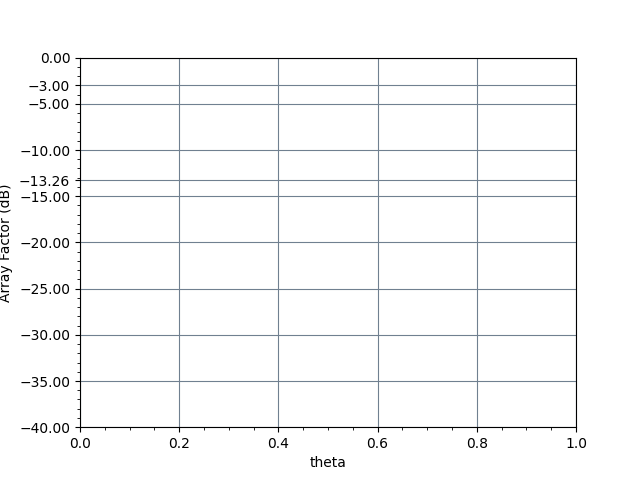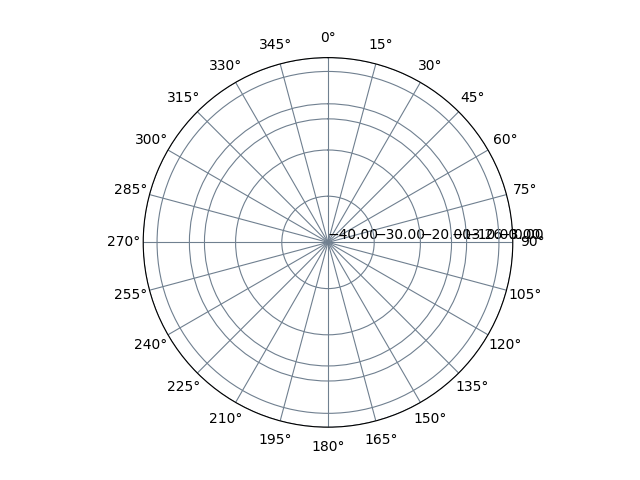# Radiation Pattern of Non-Uniform Linear Antenna Array

 Erase Graph Erase GraphOverlay Graph Graph Legend
Antenna Element -> 1 2 3 4 5 6 7 8 9 10
Weight
Progressive Phase Shift in degree
Distance from first element in $$\lambda$$

The number of elements could be reduced from the default of 10 elements by simply putting last element weights zero. For example, The 5 element array weight would be [1 1 1 1 1 0 0 0 0 0]. To introduce the progressive phase shift of 45 degrees for five elements, update the "Progressive Phase Shift in degree" section as [0 45 90 135 180 0 0 0 0 0]. The uniform distance of 0.5 $$\lambda$$ could be entered in "Distance from first element" section as [0 0.5 1.0 1.5 2 0 0 0 0 0]. The distance from first element could be also non-uniform for example [0 0.6 1.0 1.2 1.8 0 0 0 0 0]. This distance and phase for the first element is zero

Please press the "Compute" button to calculate and plot the radiation pattern of non-uniformly excited and non-uniformly spaced array

 Normalized rectangular plot YZ Cut Normalized polar plot YZ Cut## Formula

$$AF = \sum_{n=1}^{N}a_{n}e^{j(kd_{n}cos\theta +\beta )}$$
 Where: The angular wavenumber $$k=\frac{2\pi }{\lambda }$$ expresses the number of radians in a unit of distance N = number of antenna elements $$a_{n}$$ = weight of nth antenna element $$d_{n}$$ = distance of nth antenna element from first element in $$\lambda$$ $$\beta$$ = relative phase shift For the above nonuniform distance antenna array calculator the phase value of each element has to be in degrees relative to the first element
← RF Theory